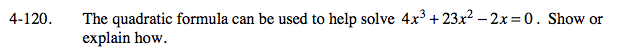### Home > A2C > Chapter 4 > Lesson 4.2.4 > Problem4-120

4-120.Factor out the x.

x(4x2 + 23x − 2) = 0

By Zero Product Property:

x = 0 or
4x2 + 23x − 2 = 0

$\textit{x}=\frac{-23\pm\sqrt{23^{2}-4(4)(-2)}}{2(4)}$